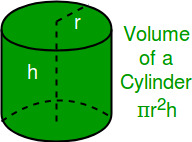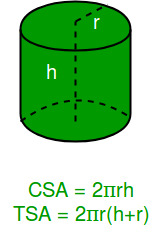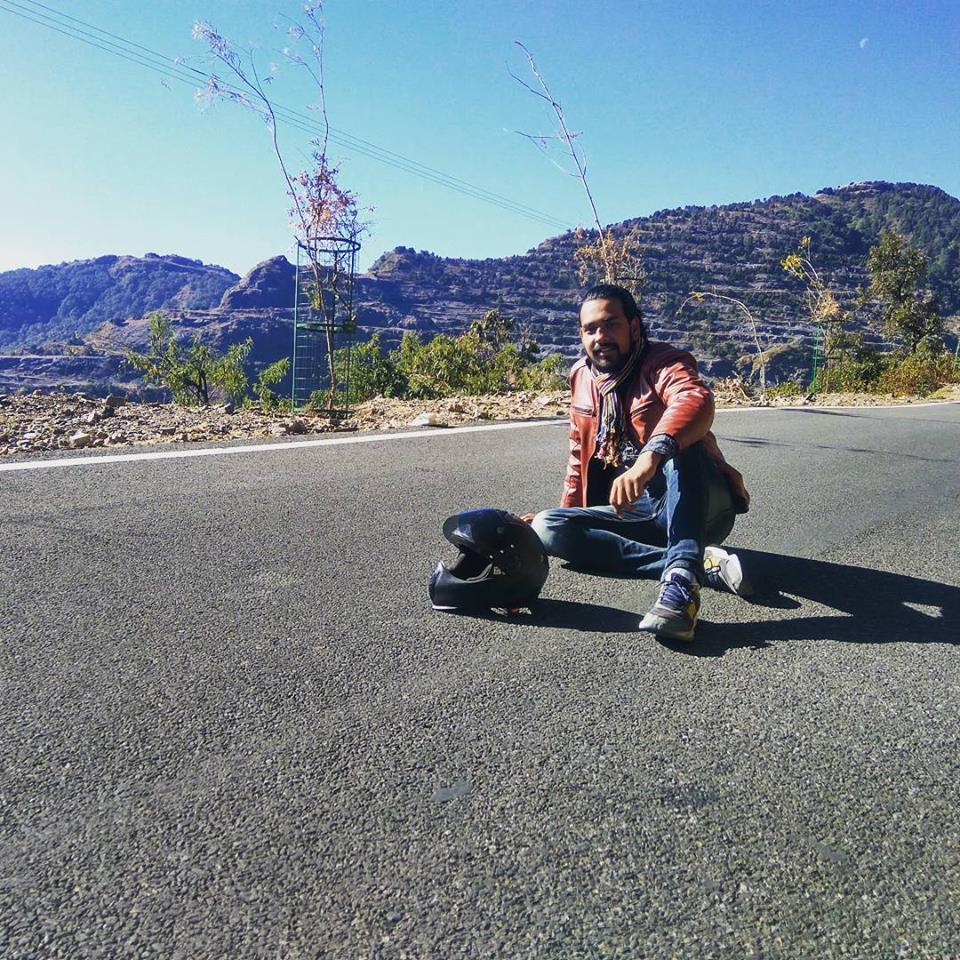# Calculate Volume, Curved Surface Area and Total Surface Area Of Cylinder

Given radius and height of Cylinder, calculate the volume, total surface area and curved surface area of cylinder.

• Volume of Cylinder:
The volume of cylinder is defined as the amount of three dimensional space occupied by the cylinder or the storage capacity of a cylinder. We can calculate volume of cylinder by using formula:where ‘ r ‘is radius of the base and ‘ h ‘ is height of cylinder.

• Total Surface Area of Cylinder:
Surface area of cylinder is the number of square units that will exactly cover outer surface of a cone. There are three surfaces in a cylinder, one curved and two circular bases. Total surface area of cylinder is the sum of the area of both circular bases and area of curved surface. The total surface area includes the area of the circular top and base, as well as the Curved Surface Area (CSA).

We can calculate curved surface area and total surface area by using formula:

•Examples:

```Input  : Enter Radius Of Cylinder   5
Enter Height Of Cylinder   8
Output : Volume       Of       Cylinder   =   628.3185307179587
Total  Surface Area  Of Cylinder =   408.4070449666731
Curved Surface Area  Of Cylinder =   251.32741228718345

Input  :Enter Radius Of Cylinder   15
Enter Height Of Cylinder   10
Output :Volume       Of       Cylinder      =   7068.583470577034
Total  Surface Area  Of Cylinder =   2356.194490192345
Curved Surface Area  Of Cylinder =   942.4777960769379
```

## C

 `#include ` `#define PI 3.14159 ` ` `  `// Function To Calculate Volume OF Cylinder ` `float` `volume(``float` `r, ``float` `h) ` `{ ` `    ``float` `vol = PI * r * r * h; ` `    ``printf``(``"Volume of Cylinder = %f \n"` `,  vol); ` `} ` ` `  `// Function To Calculate Total Surface Area OF Cylinder ` `float` `totalsurfacearea(``float` `r, ``float` `h) ` `{ ` `    ``float` `tsurf_ar = (2 * PI * r * h) + (2 * PI * r * r); ` `    ``printf``(``"Total Surface Area Of Cylinder = %f \n"` `,  tsurf_ar); ` `} ` ` `  `// Function To Calculate Curved Surface Area OF Cylinder ` `float` `curvedsurfacearea(``float` `r, ``float` `h) ` `{ ` `    ``float` `cursurf_ar = (2 * PI * r * h); ` `    ``printf``(``"Curved Surface Area Of Cylinder = %f \n"` `,  cursurf_ar); ` `} ` ` `  `// Driver Code ` `int` `main() ` `{ ` `    ``float` `r = 5; ` `    ``float` `h = 8; ` ` `  `    ``// Function Call And Printing Volume, TSA, CSA Of Cylinder ` `    ``volume(r, h); ` `    ``totalsurfacearea(r, h); ` `    ``curvedsurfacearea(r, h); ` `    ``return` `0; ` `} ` `// This code is contributed by Abhishek Agrawal. `

## C++

 `#include ` `#define PI 3.14159 ` `using` `namespace` `std; ` ` `  `// Function To Calculate Volume OF Cylinder ` `void` `volume(``float` `r, ``float` `h) ` `{ ` `    ``float` `vol = PI * r * r * h; ` `    ``cout  << ``"Volume of Cylinder =    "` `<< vol << ``"\n"` `; ` `} ` ` `  `// Function To Calculate Total Surface Area OF Cylinder ` `void` `totalsurfacearea(``float` `r, ``float` `h) ` `{ ` `    ``float` `tsurf_ar = (2 * PI * r * h) + (2 * PI * r * r); ` `    ``cout << ``"Total Surface Area Of Cylinder = "` `<< tsurf_ar << ``"\n"``; ` `} ` ` `  `// Function To Calculate Curved Surface Area OF Cylinder ` `void` `curvedsurfacearea(``float` `r, ``float` `h) ` `{ ` `    ``float` `cursurf_ar = (2 * PI * r * h); ` `    ``cout << ``"Curved Surface Area Of Cylinder = "` `           ``<< cursurf_ar << ``"\n"``; ` `} ` ` `  `// Driver code ` `int` `main() ` `{ ` `    ``float` `r = 5; ` `    ``float` `h = 8; ` `    ``volume(r, h); ` `    ``totalsurfacearea(r, h); ` `    ``curvedsurfacearea(r, h); ` `} ` `// This code is contributed by Abhishek Agrawal. `

## Java

 `// java code for illustion.... ` `import` `java.io.*; ` ` `  `class` `GFG  ` `{ ` `    ``static` `double` `PI = ``3.14159``; ` `     `  `    ``// Function To Calculate Volume OF Cylinder ` `    ``static` `void` `volume(``double` `r, ``double` `h) ` `    ``{ ` `        ``double` `vol = PI * r * r * h; ` `            ``System.out.println( ``"Volume of Cylinder = "`  `                                ``+ vol ); ` `    ``} ` `     `  `    ``// Function To Calculate Total Surface Area OF Cylinder ` `    ``static` `void` `totalsurfacearea(``double` `r, ``double` `h) ` `    ``{ ` `        ``double` `tsurf_ar = (``2` `* PI * r * h) + (``2` `* PI * r * r); ` `        ``System.out.println( ``"Total Surface Area Of Cylinder = "`  `                            ``+ tsurf_ar ); ` `    ``} ` `     `  `    ``// Function To Calculate Curved Surface Area OF Cylinder ` `    ``static` `void` `curvedsurfacearea(``double` `r, ``double` `h) ` `    ``{ ` `        ``double` `cursurf_ar = (``2` `* PI * r * h); ` `        ``System.out.println( ``"Curved Surface Area Of Cylinder = "` `                            ``+ cursurf_ar ); ` `    ``} ` ` `  `    ``// Driver code ` `    ``public` `static` `void` `main (String[] args)  ` `    ``{ ` `        ``double` `r = ``7``; ` `        ``double` `h = ``6``; ` `        ``volume(r, h); ` `        ``totalsurfacearea(r, h); ` `        ``curvedsurfacearea(r, h); ` `    ``} ` `} ` ` `  `// This code is contributed by Sunnysingh `

## Python

 `# Importing Math Library For The Value Of PI ` `import` `math ` `pi ``=` `math.pi ` ` `  `# Function To Calculate Volume OF Cylinder ` `def` `volume(r, h): ` `    ``vol ``=` `pi ``*` `r ``*` `r ``*` `h ` `    ``return` `vol ` ` `  `# Function To Calculate Total Surface Area ` `# of Cylinder ` `def` `totalsurfacearea(r, h): ` `    ``tsurf_ar ``=` `(``2` `*` `pi ``*` `r ``*` `h) ``+` `(``2` `*` `pi ``*` `r ``*` `r) ` `    ``return` `tsurf_ar ` ` `  `# Function To Calculate Curved Surface Area  ` `# of Cylinder ` `def` `curvedsurfacearea(r, h): ` `    ``cursurf_ar ``=` `(``2` `*` `pi ``*` `r ``*` `h) ` `    ``return` `cursurf_ar ` ` `  `# Driver Code ` `r ``=` `5` `h ``=` `8` `# Function Call And Printing Volume, TSA, CSA Of Cylinder ` `print``(``"Volume       Of       Cylinder   =  "``,volume(r, h)) ` `print``(``"Total  Surface Area  Of Cylinder =  "``,totalsurfacearea(r,h)) ` `print``(``"Curved Surface Area  Of Cylinder =  "``,curvedsurfacearea(r,h)) `

## C#

 `// C# code for illustion.... ` `using` `System; ` ` `  `class` `GFG { ` `     `  `    ``static` `double` `PI = 3.14159; ` `     `  `    ``// Function To Calculate Volume OF Cylinder ` `    ``static` `void` `volume(``double` `r, ``double` `h) ` `    ``{ ` `        ``double` `vol = PI * r * r * h; ` `            ``Console.WriteLine( ``"Volume of Cylinder = "` `                                ``+ vol ); ` `    ``} ` `     `  `    ``// Function To Calculate Total Surface Area ` `    ``// OF Cylinder ` `    ``static` `void` `totalsurfacearea(``double` `r, ``double` `h) ` `    ``{ ` `        ``double` `tsurf_ar = (2 * PI * r * h) +  ` `                                    ``(2 * PI * r * r); ` `        ``Console.WriteLine( ``"Total Surface Area Of "`  `                        ``+ ``"Cylinder = "` `+ tsurf_ar ); ` `    ``} ` `     `  `    ``// Function To Calculate Curved Surface Area  ` `    ``// OF Cylinder ` `    ``static` `void` `curvedsurfacearea(``double` `r, ``double` `h) ` `    ``{ ` `        ``double` `cursurf_ar = (2 * PI * r * h); ` `        ``Console.WriteLine( ``"Curved Surface Area Of"`  `                  ``+ ``" Cylinder = "` `+ cursurf_ar ); ` `    ``} ` ` `  `    ``// Driver code ` `    ``public` `static` `void` `Main ()  ` `    ``{ ` `        ``double` `r = 5; ` `        ``double` `h = 8; ` `         `  `        ``volume(r, h); ` `        ``totalsurfacearea(r, h); ` `        ``curvedsurfacearea(r, h); ` `    ``} ` `} ` ` `  `// This code is contributed by vt_m. `

## PHP

 ` `

Output:

```Volume     Of     Cylinder =  628.3185307179587
Total Surface Area Of Cylinder =  408.4070449666731
Total Surface Area Of Cylinder =  251.32741228718345```

My Personal Notes arrow_drop_upCheck out this Author's contributed articles.

If you like GeeksforGeeks and would like to contribute, you can also write an article using contribute.geeksforgeeks.org or mail your article to contribute@geeksforgeeks.org. See your article appearing on the GeeksforGeeks main page and help other Geeks.

Please Improve this article if you find anything incorrect by clicking on the "Improve Article" button below.

Improved By : vt_m, gowtham_yuvaraj